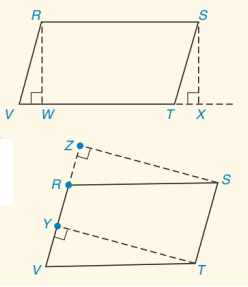Chapter 4.CT, Problem 7CT### Elementary Geometry for College St...

6th Edition
Daniel C. Alexander + 1 other
ISBN: 9781285195698

#### Solutions

Chapter
Section### Elementary Geometry for College St...

6th Edition
Daniel C. Alexander + 1 other
ISBN: 9781285195698
Textbook Problem
1 views

# Complete each statement:a) In ▭ R S T V , R W ¯ is the ________ from vertex R to base V T ¯ .b) If R W ¯ of figure (a) is congruent of T Y ¯ of figure (b), then ▭ R S T V must also bea(n)_______.To determine

(a)

To fill:

The given blanks.

Explanation

Given:

Definition:

An altitude of a parallelogram is a line segment from one vertex that is perpendicular to a non-adjacent side.

Approach:

From the figure, R is the vertex RW¯VT¯ and VT¯ is a non-adjacent side of R

To determine

(b)

To fill:

The given blanks.

### Still sussing out bartleby?

Check out a sample textbook solution.

See a sample solution

#### The Solution to Your Study Problems

Bartleby provides explanations to thousands of textbook problems written by our experts, many with advanced degrees!

Get Started

#### 2. Define the terms population, sample, parameter and statistic.

Statistics for The Behavioral Sciences (MindTap Course List)

#### Differentiate. y=sint1+tant

Single Variable Calculus: Early Transcendentals, Volume I

#### For , f(x) = 0 2 3 f(3) does not exist

Study Guide for Stewart's Single Variable Calculus: Early Transcendentals, 8th